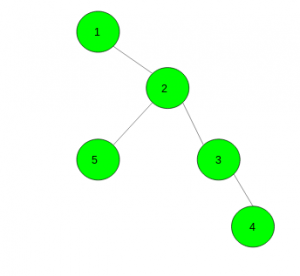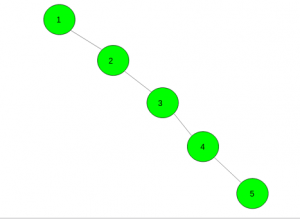# Find the number of distinct pairs of vertices which have a distance of exactly k in a tree

Given an integer k and a tree with n nodes. The task is to count the number of distinct pairs of vertices which have a distance of exactly k.

Examples:

Input: k = 2Output: 4

Input: k = 3Output: 2

## Recommended: Please try your approach on {IDE} first, before moving on to the solution.

Approach: This problem can be solved using dynamic programming. For every vertex v of the tree, we calculate values d[v][lev] (0 <= lev <= k). This value indicates the number of vertices having distance lev from v. Note that d[v] = 0.
Then we calculate the answer. For any vertex v number of pairs will be a product of the number of vertices at level j – 1 and level k – j.

Below is the implementation of the above approach:

## C++

 `// C++ implementation of the approach ` `#include ` `using` `namespace` `std; ` `#define N 5005 ` ` `  `// To store vertices and value of k ` `int` `n, k; ` ` `  `vector<``int``> gr[N]; ` ` `  `// To store number vertices at a level i ` `int` `d[N]; ` ` `  `// To store the final answer ` `int` `ans = 0; ` ` `  `// Function to add an edge between two nodes ` `void` `Add_edge(``int` `x, ``int` `y) ` `{ ` `    ``gr[x].push_back(y); ` `    ``gr[y].push_back(x); ` `} ` ` `  `// Function to find the number of distinct ` `// pairs of the vertices which have a distance ` `// of exactly k in a tree ` `void` `dfs(``int` `v, ``int` `par) ` `{ ` `    ``// At level zero vertex itself is counted ` `    ``d[v] = 1; ` `    ``for` `(``auto` `i : gr[v]) { ` `        ``if` `(i != par) { ` `            ``dfs(i, v); ` ` `  `            ``// Count the pair of vertices at  ` `            ``// distance k ` `            ``for` `(``int` `j = 1; j <= k; j++) ` `                ``ans += d[i][j - 1] * d[v][k - j]; ` ` `  `            ``// For all levels count vertices ` `            ``for` `(``int` `j = 1; j <= k; j++) ` `                ``d[v][j] += d[i][j - 1]; ` `        ``} ` `    ``} ` `} ` ` `  `// Driver code ` `int` `main() ` `{ ` `    ``n = 5, k = 2; ` ` `  `    ``// Add edges ` `    ``Add_edge(1, 2); ` `    ``Add_edge(2, 3); ` `    ``Add_edge(3, 4); ` `    ``Add_edge(2, 5); ` ` `  `    ``// Function call ` `    ``dfs(1, 0); ` ` `  `    ``// Required answer ` `    ``cout << ans; ` ` `  `    ``return` `0; ` `} `

## Java

 `// Java implementation of the approach ` `import` `java.util.*; ` ` `  `class` `GFG  ` `{ ` `    ``static` `final` `int` `N = ``5005``; ` ` `  `    ``// To store vertices and value of k ` `    ``static` `int` `n, k; ` ` `  `    ``static` `Vector[] gr = ``new` `Vector[N]; ` ` `  `    ``// To store number vertices at a level i ` `    ``static` `int``[][] d = ``new` `int``[N][``505``]; ` ` `  `    ``// To store the final answer ` `    ``static` `int` `ans = ``0``; ` ` `  `    ``// Function to add an edge between two nodes ` `    ``static` `void` `Add_edge(``int` `x, ``int` `y) ` `    ``{ ` `        ``gr[x].add(y); ` `        ``gr[y].add(x); ` `    ``} ` ` `  `    ``// Function to find the number of distinct ` `    ``// pairs of the vertices which have a distance ` `    ``// of exactly k in a tree ` `    ``static` `void` `dfs(``int` `v, ``int` `par)  ` `    ``{ ` `        ``// At level zero vertex itself is counted ` `        ``d[v][``0``] = ``1``; ` `        ``for` `(Integer i : gr[v]) ` `        ``{ ` `            ``if` `(i != par) ` `            ``{ ` `                ``dfs(i, v); ` ` `  `                ``// Count the pair of vertices at ` `                ``// distance k ` `                ``for` `(``int` `j = ``1``; j <= k; j++) ` `                    ``ans += d[i][j - ``1``] * d[v][k - j]; ` ` `  `                ``// For all levels count vertices ` `                ``for` `(``int` `j = ``1``; j <= k; j++) ` `                    ``d[v][j] += d[i][j - ``1``]; ` `            ``} ` `        ``} ` `    ``} ` ` `  `    ``// Driver code ` `    ``public` `static` `void` `main(String[] args) ` `    ``{ ` `        ``n = ``5``; ` `        ``k = ``2``; ` `        ``for` `(``int` `i = ``0``; i < N; i++) ` `            ``gr[i] = ``new` `Vector(); ` `         `  `        ``// Add edges ` `        ``Add_edge(``1``, ``2``); ` `        ``Add_edge(``2``, ``3``); ` `        ``Add_edge(``3``, ``4``); ` `        ``Add_edge(``2``, ``5``); ` ` `  `        ``// Function call ` `        ``dfs(``1``, ``0``); ` ` `  `        ``// Required answer ` `        ``System.out.print(ans); ` ` `  `    ``} ` `} ` ` `  `// This code is contributed by PrinciRaj1992 `

## Python3

 `# Python3 implementation of the approach ` `N ``=` `5005` ` `  `# To store vertices and value of k ` `n, k ``=` `0``, ``0` ` `  `gr ``=` `[[] ``for` `i ``in` `range``(N)] ` ` `  `# To store number vertices at a level i ` `d ``=` `[[``0` `for` `i ``in` `range``(``505``)]  ` `        ``for` `i ``in` `range``(N)] ` ` `  `# To store the final answer ` `ans ``=` `0` ` `  `# Function to add an edge between two nodes ` `def` `Add_edge(x, y): ` `    ``gr[x].append(y) ` `    ``gr[y].append(x) ` ` `  `# Function to find the number of distinct ` `# pairs of the vertices which have a distance ` `# of exactly k in a tree ` `def` `dfs(v, par): ` `    ``global` `ans ` `     `  `    ``# At level zero vertex itself is counted ` `    ``d[v][``0``] ``=` `1` `    ``for` `i ``in` `gr[v]: ` `        ``if` `(i !``=` `par): ` `            ``dfs(i, v) ` ` `  `            ``# Count the pair of vertices at ` `            ``# distance k ` `            ``for` `j ``in` `range``(``1``, k ``+` `1``): ` `                ``ans ``+``=` `d[i][j ``-` `1``] ``*` `d[v][k ``-` `j] ` ` `  `            ``# For all levels count vertices ` `            ``for` `j ``in` `range``(``1``, k ``+` `1``): ` `                ``d[v][j] ``+``=` `d[i][j ``-` `1``] ` ` `  `# Driver code ` `n ``=` `5` `k ``=` `2` ` `  `# Add edges ` `Add_edge(``1``, ``2``) ` `Add_edge(``2``, ``3``) ` `Add_edge(``3``, ``4``) ` `Add_edge(``2``, ``5``) ` ` `  `# Function call ` `dfs(``1``, ``0``) ` ` `  `# Required answer ` `print``(ans) ` ` `  `# This code is contributed by Mohit Kumar `

## C#

 `// C# implementation of the approach ` `using` `System; ` `using` `System.Collections.Generic; ` ` `  `class` `GFG  ` `{ ` `    ``static` `readonly` `int` `N = 5005; ` ` `  `    ``// To store vertices and value of k ` `    ``static` `int` `n, k; ` ` `  `    ``static` `List<``int``>[] gr = ``new` `List<``int``>[N]; ` ` `  `    ``// To store number vertices at a level i ` `    ``static` `int``[,] d = ``new` `int``[N, 505]; ` ` `  `    ``// To store the readonly answer ` `    ``static` `int` `ans = 0; ` ` `  `    ``// Function to add an edge between two nodes ` `    ``static` `void` `Add_edge(``int` `x, ``int` `y) ` `    ``{ ` `        ``gr[x].Add(y); ` `        ``gr[y].Add(x); ` `    ``} ` ` `  `    ``// Function to find the number of distinct ` `    ``// pairs of the vertices which have a distance ` `    ``// of exactly k in a tree ` `    ``static` `void` `dfs(``int` `v, ``int` `par)  ` `    ``{ ` `        ``// At level zero vertex itself is counted ` `        ``d[v, 0] = 1; ` `        ``foreach` `(``int` `i ``in` `gr[v]) ` `        ``{ ` `            ``if` `(i != par) ` `            ``{ ` `                ``dfs(i, v); ` ` `  `                ``// Count the pair of vertices at ` `                ``// distance k ` `                ``for` `(``int` `j = 1; j <= k; j++) ` `                    ``ans += d[i, j - 1] * d[v, k - j]; ` ` `  `                ``// For all levels count vertices ` `                ``for` `(``int` `j = 1; j <= k; j++) ` `                    ``d[v, j] += d[i, j - 1]; ` `            ``} ` `        ``} ` `    ``} ` ` `  `    ``// Driver code ` `    ``public` `static` `void` `Main(String[] args) ` `    ``{ ` `        ``n = 5; ` `        ``k = 2; ` `        ``for` `(``int` `i = 0; i < N; i++) ` `            ``gr[i] = ``new` `List<``int``>(); ` `         `  `        ``// Add edges ` `        ``Add_edge(1, 2); ` `        ``Add_edge(2, 3); ` `        ``Add_edge(3, 4); ` `        ``Add_edge(2, 5); ` ` `  `        ``// Function call ` `        ``dfs(1, 0); ` ` `  `        ``// Required answer ` `        ``Console.Write(ans); ` ` `  `    ``} ` `} ` ` `  `// This code is contributed by Rajput-Ji `

## PHP

 ` `

Output:

```4
```My Personal Notes arrow_drop_upCheck out this Author's contributed articles.

If you like GeeksforGeeks and would like to contribute, you can also write an article using contribute.geeksforgeeks.org or mail your article to contribute@geeksforgeeks.org. See your article appearing on the GeeksforGeeks main page and help other Geeks.

Please Improve this article if you find anything incorrect by clicking on the "Improve Article" button below.